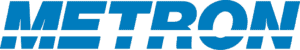## Introduction to Nonlinear Gradient Descent

Overview

Nonlinear gradient descent methods are useful for finding the minimum value of a differentiable function f(x). By flipping the sign of the function, they can also find the maximum value. In that context, they are sometimes called hill climbing algorithms. They can be applied to a wide range of problems. When used to optimize the locations of a set of objects to satisfy a joint goal, x is relatively low-dimensional, say up to fifty. When used to optimize the set of weights in a neural network, x can have a million dimensions. As the dimension grows, the time required to find the minimum also grows.

Methods

Descent methods start at a given point x0, and take a sequence of steps to reach the optimal point x*. Each step is designed to decrease the value of f(x). This is sometimes called walking the surface to try to reach the bottom. Computing the functional form of the gradient of f is the best way to choose the step direction. If that cannot be done, then sampling methods can be used. Those involve trying different step directions and choosing the one that decreases f the most.

Challenges and Alternatives

Depending on the shape of f, choosing the steepest descent direction may cause the method to get stuck in a local minimum. Instead of reaching the bottom of the hill, the walker gets stuck in a shallow pit along the way. Stochastic gradient descent methods address this problem by taking random steps occasionally to get out of these local minima. The balance between choosing the steepest descent direction and a random direction depends on the method used.